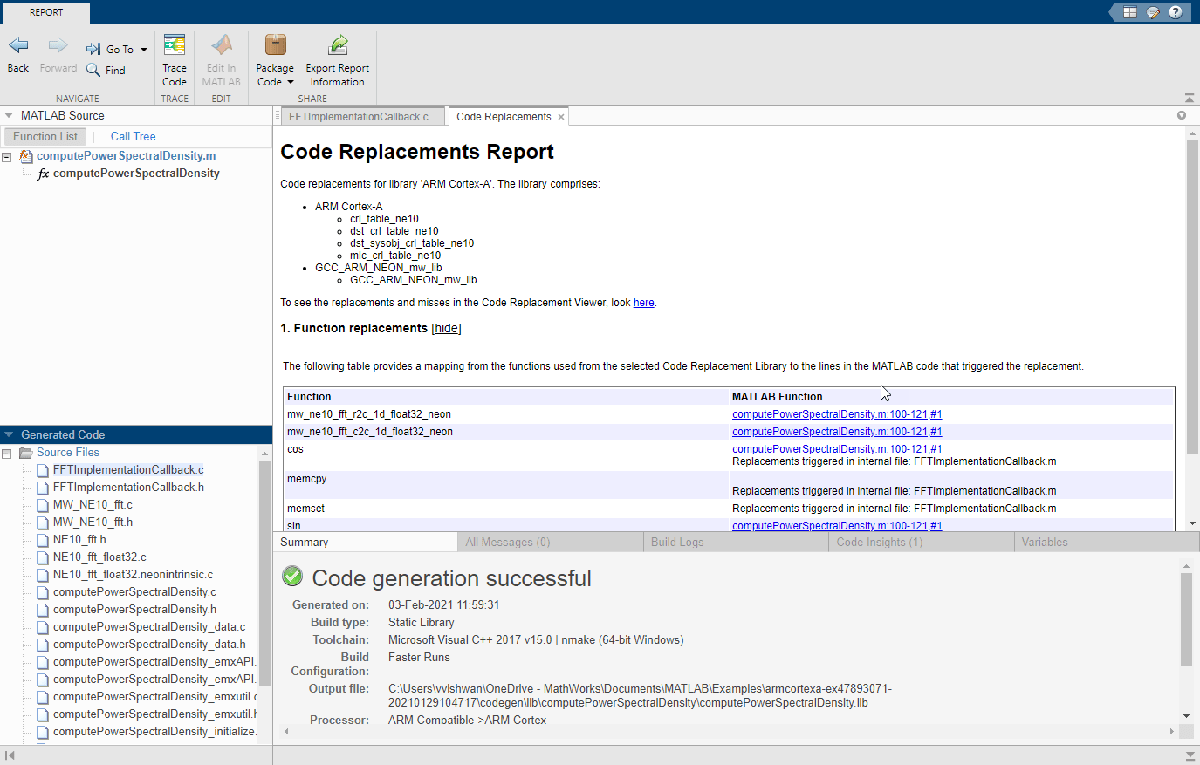# FFT for Spectral Analysis on ARM Cortex-A Processor

This example shows how to use Embedded Coder® Support Package for ARM® Cortex®-A processors to generate optimized C code for the FFT function for spectral analysis with the NE10 library. This example also shows how to use the QEMU ARM Cortex-A9 emulator to verify the generated code. For more details on the FFT function for spectral analysis, see the Fourier Transforms.

### Introduction

This example uses the concept of the FFT function for spectral analysis to show how to generate optimized C code for an ARM Cortex-A processor. A common use of FFTs is to find the frequency components of a signal buried in a noisy time-domain signal. For more information, see the `fft`` `function.

The MATLAB® function `computePowerSpectralDensity` computes the power spectral density of the input array signal (`noisySignal`) for an n-point transform length (`nfft`). The `fft` function computes the discrete Fourier transform (DFT) of `noisySignal` by using a fast Fourier transform (FFT) algorithm. For details about the power spectral density, see the Power Spectral Density Estimates Using FFT (Signal Processing Toolbox).

```function Pyy = computePowerSpectralDensity(noisySignal,nfft) Y = fft(noisySignal,nfft); % Find the FFT of the Noisy Signal Pyy = Y.*conj(Y)/nfft; % Compute the Power Spectral Density using the complex conjugate end ```

Using the Code Replacement Library (CRL), you can generate optimized C code for computing power spectral density function. You can verify the generated code using the Processor-in-the-Loop (PIL) workflow on the QEMU ARM Cortex-A9 emulator. For more information on PIL, see Code Verification Through Software-in-the-Loop and Processor-in-the-Loop Execution. For more information on the QEMU emulator, see the QEMU website.

The Embedded Coder Support Package for ARM Cortex-A processor also supports code generation for these cases:

• Input array signal length (`noisySignal`) not equal to n-point transform length (`nfft`)

• Variable-sized input signal

• N-dimensional input signal

• Non-Power-of-2 input signal

### Use Case 1 : Non-Power-of-2 Input Signal Code Generation

This use case has an FFT input signal that is of fixed size and equal to the n-point transform length. The length of the input signal is a non-power-of-2. To generate C code, follow these steps.

1. Create an input signal for the FFT calculation.

```freq = 1000; % Sampling frequency t = 0:1/freq:0.25; % Time axis data = single(sin(2*pi*50*t) + sin(2*pi*120*t)); % Data has waves of frequency 50Hz & 120Hz noise = single(2*randn(size(t))); % Noise data noisySignal = data + noise; % Noisy signal of length 251 nfft = length(noisySignal); % n-point transform of 251 ```

2. Create a code generation configuration object for generating a C/C++ static library.

``` cfg = coder.config('lib'); cfg.CodeReplacementLibrary = 'ARM Cortex-A'; cfg.GenerateCodeReplacementReport = true; cfg.TargetLangStandard = 'C89/C90 (ANSI)'; cfg.HardwareImplementation.ProdHWDeviceType = 'ARM Compatible->ARM Cortex'; cfg.GenCodeOnly = true; ```

3. Generate C code for the MATLAB function `computePowerSpectralDensity` by using the `codegen` command.

``` codegen computePowerSpectralDensity -config cfg -args {noisySignal,nfft} -launchreport ```

4. Check the report and the generated code. This figure shows the Code replacement report.5. Before verifying the generated code, change the code configuration object to run on a QEMU ARM Cortex-A9 emulator.

``` cfg.GenCodeOnly = false; cfg.VerificationMode = 'PIL'; hw = coder.hardware('ARM Cortex-A9 (QEMU)'); cfg.Hardware = hw; cfg.StackUsageMax = 1024; ```

6. Verify the generated code with the PIL.

``` codegen computePowerSpectralDensity -args {noisySignal,nfft} -config cfg -report yTargetPIL = computePowerSpectralDensity_pil(noisySignal,nfft); ```

### Use Case 2 : Variable Input Signal Code Generation

This use case has an FFT input signal of variable size that uses dynamic memory allocation. To generate C code, follow these steps.

1. Create a code generation configuration object and generate C code for the MATLAB function `computePowerSpectralDensity` by using the `codegen` command.

```cfg = coder.config('lib'); cfg.CodeReplacementLibrary = 'ARM Cortex-A'; cfg.GenerateCodeReplacementReport = true; cfg.TargetLangStandard = 'C89/C90 (ANSI)'; cfg.HardwareImplementation.ProdHWDeviceType = 'ARM Compatible->ARM Cortex'; cfg.GenCodeOnly = true; codegen computePowerSpectralDensity -config cfg -args {coder.typeof(single(1),[1 inf]),coder.typeof(1)} -report ```

2. Before verifying the generated code, change the code configuration object to run on a QEMU ARM Cortex-A9 emulator. Verify the generated code with the PIL by using a different FFT input signal and n-point transform length.

```cfg.GenCodeOnly = false; cfg.VerificationMode = 'PIL'; hw = coder.hardware('ARM Cortex-A9 (QEMU)'); cfg.Hardware = hw; cfg.StackUsageMax = 1024; codegen computePowerSpectralDensity -config cfg -args {coder.typeof(single(1),[1 inf]),coder.typeof(1)} -report ```
``` noisySignal = rand(1,167,'single'); nfft = 221; yTargetPILVarSize = computePowerSpectralDensity_pil(noisySignal,nfft); ```

### Use Case 3 : N-Dimensional Array Input Signal Code Generation

This use case has an input signal that is an N-dimensional array and has an FFT input signal that is not equal to n-point transform length. To generate C code, follow these steps.

1. Create a code generation configuration object and generate C code for the MATLAB function `computePowerSpectralDensity` for a two-dimensional array input.

``` cfg = coder.config('lib'); cfg.CodeReplacementLibrary = 'ARM Cortex-A'; cfg.GenerateCodeReplacementReport = true; cfg.TargetLangStandard = 'C89/C90 (ANSI)'; cfg.HardwareImplementation.ProdHWDeviceType = 'ARM Compatible->ARM Cortex'; cfg.VerificationMode = 'PIL'; hw = coder.hardware('ARM Cortex-A9 (QEMU)'); cfg.Hardware = hw; cfg.StackUsageMax = 1024; codegen computePowerSpectralDensity -config cfg -args {coder.typeof(single(1),[100 2]),coder.Constant(100)} -report ```

2. Verify the generated code with the PIL.

``` noisySignal = rand(100,2,'single'); nfft = 100; yTargetPILVarSize1 = computePowerSpectralDensity_pil(noisySignal,nfft); ```